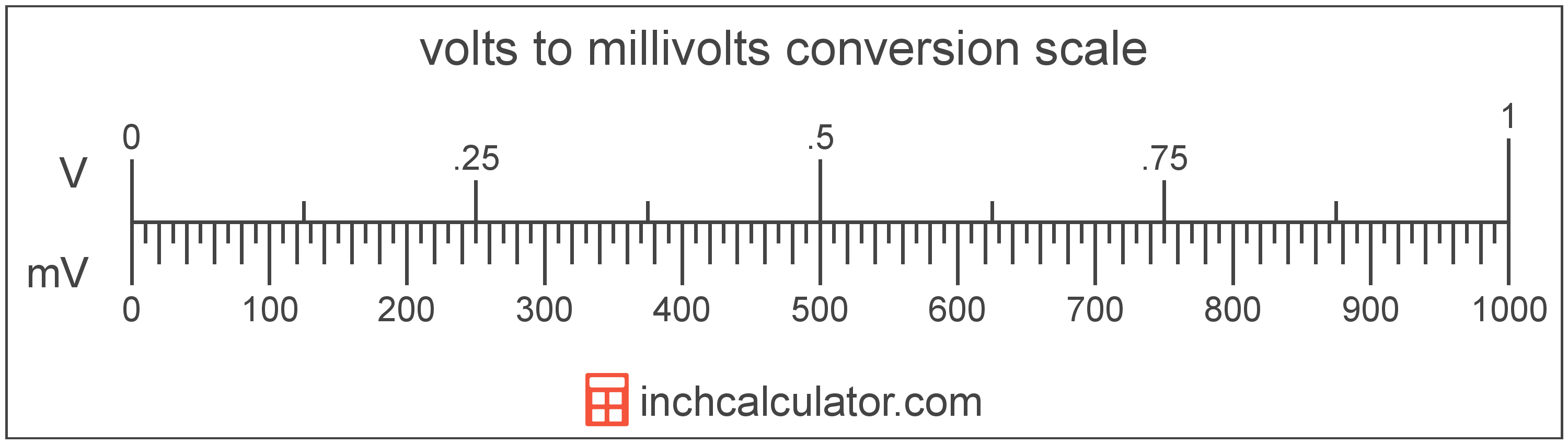# Millivolts to Volts Converter

Enter the voltage in millivolts below to get the value converted to volts.

Results in Volts:1 mV = 0.001 V

Do you want to convert volts to millivolts?

## How to Convert Millivolts to Volts

To convert a measurement in millivolts to a measurement in volts, divide the voltage by the following conversion ratio: 1,000 millivolts/volt.

Since one volt is equal to 1,000 millivolts, you can use this simple formula to convert:

volts = millivolts ÷ 1,000

The voltage in volts is equal to the voltage in millivolts divided by 1,000.

For example, here's how to convert 5,000 millivolts to volts using the formula above.
volts = (5,000 mV ÷ 1,000) = 5 V## What Is a Millivolt?

One millivolt is equal to 1/1,000 of a volt, which is the potential difference that would move one ampere of current against one ohm of resistance.

The millivolt is a multiple of the volt, which is the SI derived unit for voltage. In the metric system, "milli" is the prefix for thousandths, or 10-3. Millivolts can be abbreviated as mV; for example, 1 millivolt can be written as 1 mV.

## What Is a Volt?

One volt is equal to the potential difference that would move one ampere of current against one ohm of resistance.

The volt is the SI derived unit for voltage in the metric system. Volts can be abbreviated as V; for example, 1 volt can be written as 1 V.

### Voltage and Ohm's Law

Voltage is a measurement of electromotive force and the electrical potential difference between two points of a conductor.

Ohm's Law states the current between two points on a conductor is proportional to the voltage and inversely proportional to the resistance. Using Ohm's Law, it's possible to express the potential difference in volts as an expression using current and resistance.

VV = IA × R

The potential difference in volts is equal to the current in amperes times the resistance in ohms.

## Millivolt to Volt Conversion Table

Table showing various millivolt measurements converted to volts.
Millivolts Volts
1 mV 0.001 V
2 mV 0.002 V
3 mV 0.003 V
4 mV 0.004 V
5 mV 0.005 V
6 mV 0.006 V
7 mV 0.007 V
8 mV 0.008 V
9 mV 0.009 V
10 mV 0.01 V
20 mV 0.02 V
30 mV 0.03 V
40 mV 0.04 V
50 mV 0.05 V
60 mV 0.06 V
70 mV 0.07 V
80 mV 0.08 V
90 mV 0.09 V
100 mV 0.1 V
200 mV 0.2 V
300 mV 0.3 V
400 mV 0.4 V
500 mV 0.5 V
600 mV 0.6 V
700 mV 0.7 V
800 mV 0.8 V
900 mV 0.9 V
1,000 mV 1 V

## References

1. International Bureau of Weights and Measures, The International System of Units, 9th Edition, 2019, https://www.bipm.org/documents/20126/41483022/SI-Brochure-9-EN.pdf